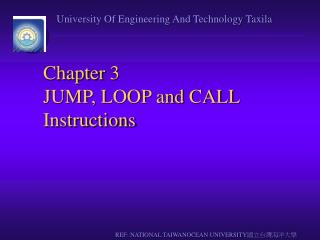DownloadDownload PresentationChapter 3 JUMP, LOOP and CALL Instructions

# Chapter 3 JUMP, LOOP and CALL Instructions

Download Presentation## Chapter 3 JUMP, LOOP and CALL Instructions

- - - - - - - - - - - - - - - - - - - - - - - - - - - E N D - - - - - - - - - - - - - - - - - - - - - - - - - - -
##### Presentation Transcript

1. Chapter 3JUMP, LOOP and CALL Instructions REF::NATIONAL TAIWANOCEAN UNIVERSITY國立台灣海洋大學

2. Outlines • Loop instructions • Conditional jump instructions • Conditions determining conditional jump • Unconditional long & short jumps • Calculate target addresses for jumps • Subroutines • Using stack in subroutines • Crystal frequency vs. machine cycle • Code programs to generate time delay

3. Looping

4. Loop inside a Loop (Nested Loop)

5. 8051 Conditional Jump Instructions

6. Conditional Jump Example

7. Conditional Jump Example

8. Unconditional Jump Instructions • All conditional jumps are short jumps • Target address within -128 to +127 of PC • LJMP (long jump): 3-byte instruction • 2-byte target address: 0000 to FFFFH • Original 8051 has only 4KB on-chip ROM • SJMP (short jump): 2-byte instruction • 1-byte relative address: -128 to +127

9. Call Instructions • LCALL (long call): 3-byte instruction • 2-byte address • Target address within 64K-byte range • ACALL (absolute call): 2-byte instruction • 11-bit address • Target address within 2K-byte range

10. LCALL

11. CALL Instruction & Role of Stack

12. CALL Instruction & Role of Stack

13. Calling Subroutines

14. Calling Subroutines

15. ACALL (absolute call)

16. Programming Efficiently

17. Time Delay Generation & Calculation • 1 instruction = n machine cycle • 1 machine cycle = 12 clock cycles

18. Delay Calculation

19. Delay Calculation Example

20. Delay Calculation Example

21. Increasing Delay Using NOP

22. Large Delay Using Nested Loop

23. Chapter 6Arithmetic Instructions and Programs REF::NATIONAL TAIWANOCEAN UNIVERSITY國立台灣海洋大學

24. Outlines • Range of numbers in 8051 unsigned data • Addition & subtraction instructions for unsigned data • BCD system of data representation • Packed and unpacked BCD data • Addition & subtraction on BCD data • Range of numbers in 8051 signed data • Signed data arithmetic instructions • Carry & overflow problems & corrections

25. Addition of Unsigned Numbers • ADD A, source ; A = A + source

26. ADDC & Addition of 16-bit Numbers 1 3C E7 3B 8D 78 74 +

27. BCD Number System • Unpacked BCD: 1 byte • Packed BCD: 4 bits

28. Adding BCD Numbers & DA Instruction MOV A,#17H ADD A,#28H MOV A,#47H ;A=47H first BCD operand MOV B,#25H ;B=25 second BCD operand ADD A,B ;hex (binary) addition (A=6CH) DA A ;adjust for BCD addition (A=72H) HEX BCD 29 0010 1001 + 18 + 0001 1000 41 0100 0001 AC=1 + 6 + 0110 47 0100 0111

29. Subtraction of Unsigned Numbers • SUBB A, source ; A = A – source – CY • SUBB when CY = 0 • Take 2’s complement of subtraend (source) • Add it to minuend • Invert carry

30. Example (Positive Result)

31. Example (Negative Result)

32. SUBB When CY = 1 • For multibyte numbers

33. Multiplication of Unsigned Numbers • MUL AB ; A  B, place 16-bit result in B and A MOV A,#25H ;load 25H to reg. A MOV B,#65H ;load 65H in reg. B MUL AB ;25H * 65H = E99 where ;B = 0EH and A = 99H

34. Division of Unsigned Numbers • DIV AB ; divide A by B MOV A,#95H ;load 95 into A MOV B,#10H ;load 10 into B DIV AB ;now A = 09 (quotient) and ;B = 05 (remainder)

35. Example ( 1 of 2 )

36. Example ( 2 of 2 )

37. Signed 8-bit Operands • Covert to 2’s complement • Write magnitude of number in 8-bit binary (no sign) • Invert each bit • Add 1 to it

38. Example

39. Example

40. Example

41. Byte-sized Signed Numbers Ranges Decimal Binary Hex -128 1000 0000 80 -127 1000 0001 81 -126 1000 0010 82 …. ………… .. -2 1111 1110 FE -1 1111 1111 FF 0 0000 0000 00 +1 0000 0001 01 +2 0000 0010 02 … ………… ... +127 0111 1111 7F

42. Overflow in Signed Number Operations

43. When Is the OV Flag Set? • Either: there is a carry from D6 to D7 but no carry out of D7 (CY = 0) • Or: there is a carry from D7 out (CY = 1) but no carry from D6 to D7

44. Example

45. Example

46. Example

47. Chapter 7LOGIC INSTRUCTIONS AND PROGRAMS REF::NATIONAL TAIWANOCEAN UNIVERSITY國立台灣海洋大學

48. Define the truth tables for logic functions AND, OR, XOR Code 8051 Assembly language logic function instructions Use 8051 logic instructions for bit manipulation Use compare and jump instructions for program control Code 8051 rotate and swap instructions Code 8051 programs for ASCII and BCD data conversion Outlines

49. OR ORL destination, source ;dest = dest OR source